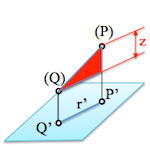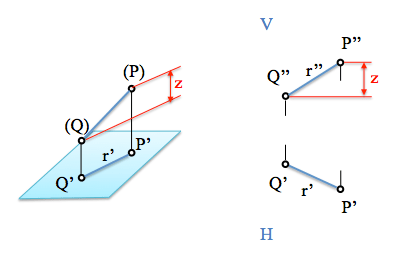# Diédrico System: True magnitude of the lineWhen projecting a straight orthogonal projection on a plane, its projection, generally, is smaller than the original measure.

Given a straight (segment bounded by two points) we want to determine its true magnitude and the angle it makes with the planes of projection.

Overall measurement problems are reduced to the application of the Pythagorean theorem, being the determination of the hypotenuse of a right triangle in order for the solution to these problems.

Analysis in figure one can see that the distance between points P and Q is the hypotenuse of a right triangle whose legs height difference (z) between the two points and the projection, r’, on the plane perpendicular to that direction used to obtain the coordinates.The projection r’ get on the horizontal plane, while the difference in height, z, be seen in the vertical plane of projection. To obtain the true magnitude construct the triangle coupling data of the projections, in this case the horizontal.Note that it is obtained by the angle between the line with one of the planes of projection, particularly with respect to the coordinate z we.

Similarly, it would have solved the problem with the projection r” but using, instead of the z dimension, the difference between swings from the vertical plane (and), therefore obtaining the angle of the line with this plane.

How calcularías the angle from the vertical plane? Could you adapt the necessary construction?